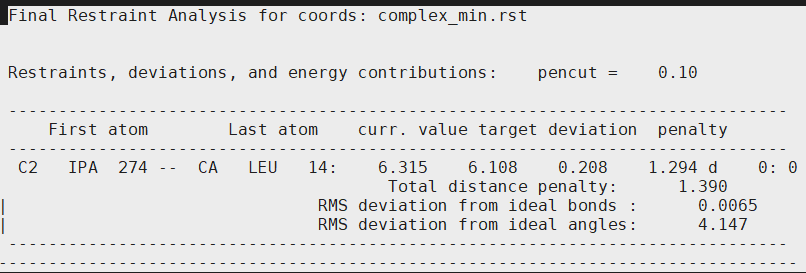# Re: [AMBER] NMR restraints

From: Debarati DasGupta <debarati_dasgupta.hotmail.com>
Date: Mon, 2 Nov 2020 22:21:27 +0000

From: Debarati DasGupta<mailto:debarati_dasgupta.hotmail.com>
Sent: 01 November 2020 09:12
To: David A Case<mailto:david.case.rutgers.edu>
Subject: RE: [AMBER] NMR restraints

Hello Prof. Case,
So I did run a simple 10 step minimization ( input file Minimization:-
&cntrl
imin = 1, ntmin = 2,
maxcyc=10,
ntpr = 1, ntwe = 1, ntwx =1,
ntave=1,
iwrap = 1,
nsnb = 10,
ntb = 1,
ioutfm=1,
icfe = 1, ifsc = 1, clambda=0.00922, scalpha = 0.5, scbeta = 12.0,
nmropt=1,
/
&wt type='DUMPFREQ', istep1=1, /
&wt type='END', /
DISANG=output.disang
DUMPAVE=dist_0.00922.dat
LISTIN=POUT
LISTOUT=POUT)

This is the contents of the min.out file attached in the email.
What I am a bit perplexed is the contents of the last part of the output file:
[cid:image001.png.01D6B02E.50730DF0]

The restraints, deviations, and energy contributions are only listed for (C2 IPA and CA LEU)! I had 3 distance restraints in my output.disang ( 3 CA atoms of my kinase with the C2 atom of isopropanol)
Output.disang contents pasted here:

&rst iat=4396,204,0
r1=5.607614, r2=6.107614, r3=6.107614, r4=6.607614, rk2=30.000000, rk3=30.000000,
nstep1=0, nstep2=0,
&end
&rst iat=4396,2132,0
r1=6.332594, r2=6.832594, r3=6.832594, r4=7.332594, rk2=30.000000, rk3=30.000000,
nstep1=0, nstep2=0,
&end
&rst iat=4396,1387,0
r1=5.165932, r2=5.665932, r3=5.665932, r4=6.165932, rk2=30.000000, rk3=30.000000,
nstep1=0, nstep2=0,
&end

What is the reason you think that the rms deviations and penalties are only being calculated for one pair ( LEU CA atom and C2 of IPA; why not the other pairs)
Am I making a mistake in sketching the format of the disang file?
I am running a simple minimization now without TI section and shall update you in a moment.
I used cpptraj to create it from my prmtop and reference was my starting complex.pdb file
Thanks
Debarati

From: David A Case<mailto:david.case.rutgers.edu>
Sent: 01 November 2020 08:41
To: Debarati DasGupta<mailto:debarati_dasgupta.hotmail.com>
Subject: Re: [AMBER] NMR restraints

On Sat, Oct 31, 2020, Debarati DasGupta wrote:

>I just ran a 100 step minimization to see how the NMR restraints are
>applied.
>So in the min.out file; after the part where the TI masks are read, I
>found this section on restraints.
>Is this getting printed out on the out file because I have set LISTIN=POUT?

Yes. It looks like you should be getting non-zero NMR energies. Run a
1-step minimization, and study both the LISTIN (inoming) and LISTOUT
(final) sections carefully. Try without TI to see that you can get the
energies you want from the simplest calculation, in particular, with no
softcore region. [Interactions between softcore and non-softcore atoms
are treated in a special way, but I'm reaching here.]

The main goal is to find a simple calculation where the NMR energies are
what you expect, then slowly morph things to the calculation you want,
in order to pin down the problem. None of these tests should require
more than just looking at what happens at the first point. You could
even run a zero-step MD just to see if there could be some discrepancy
between minimization and MD.

....dac

_______________________________________________
AMBER mailing list
AMBER.ambermd.org
http://lists.ambermd.org/mailman/listinfo/amber(image/png attachment: 86957F56C1EA408C871BEA3A50EE4331.png)

Received on Mon Nov 02 2020 - 14:30:03 PST
Custom Search